# Cellular algebra

(diff) ← Older revision | Latest revision (diff) | Newer revision → (diff)

(in algebraic combinatorics)

Algebras introduced by B.Yu. Weisfeiler and A.A. Leman [a9] and initially studied by representatives of the Soviet school of algebraic combinatorics. The first stage of this development was summarized in [a8]. Important particular examples of cellular algebras are the coherent algebras (cf. also Coherent algebra).

A cellular algebraof orderand rankis a matrix subalgebra of the full matrix algebra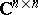of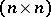-matrices oversuch that:is closed with respect to the Hermitian adjoint;, where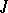is the all-one matrix;is closed with respect to Schur–Hadamard multiplication (cf. also Coherent algebra). A coherent algebra is a cellular algebra that contains the unit matrix.

Like coherent algebras, a cellular algebrahas a unique standard basis of zero-one matrices, consisting of mutually orthogonal Schur–Hadamard idempotents. The notation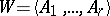indicates thathas the standard basis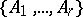.

A cellular algebrais called a cell if the all unit matrixbelongs to its centre (cf. also Centre of a ring). Cells containing the unit matrixare equivalent to Bose–Mesner algebras. If the entries of the matrices inare restricted to the ring, then the corresponding ring of matrices is called a cellular ring.

The relational analogue of cellular algebras with the unit matrixwas introduced by D.G. Higman in [a3] under the name coherent configuration. For a long time the theories of cellular algebras and coherent configurations were developed relatively independently. After the appearance of Higman's paper [a4], where the terminology of coherent algebras was coined, most researchers switched to the terminology of coherent algebras.

As a rule, only cellular algebras containing(that is, coherent algebras) were investigated. Situations where cellular algebras are required properly appear rarely, see for example [a7], where a particular kind of such algebras are treated as pseudo-Schur rings.

The initial motivation for the introduction of cellular algebras was the graph isomorphism problem (cf. also Graph isomorphism).

The intersection of cellular algebras is again a cellular algebra. For each set of matrices of the same orderit is possible to determine a minimal cellular algebra containing this set. In particular, ifis an-vertex graph and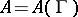is its adjacency matrix, then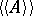denotes the smallest cellular algebra containing. It is called the cellular closure (or Weisfeiler–Leman closure) of.

In [a9] and [a8], Weisfeiler and Leman described an algorithm of stabilization which has an inputand returnsin polynomial time, depending on. Isomorphic graphs have isomorphic cellular closures, and this fact has important applications, see [a6]. In general,does not coincide with the centralizer algebra of the automorphism group of the graph; however, they do coincide for many classes of graphs, for example for the algebraic forests introduced in [a1].

For each cellular algebraone can introduce its automorphism groupHere,is the automorphism group of the graph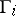with adjacency matrix. For each permutation group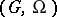, its centralizer algebrais a cellular algebra with matrix. Thus, the functorsandintroduce a Galois correspondence between cellular (coherent) algebras and permutation groups. Some properties and applications of this correspondence are considered in [a2], [a5].

How to Cite This Entry:
Cellular algebra. Encyclopedia of Mathematics. URL: http://encyclopediaofmath.org/index.php?title=Cellular_algebra&oldid=13370
This article was adapted from an original article by Mikhail Klin (originator), which appeared in Encyclopedia of Mathematics - ISBN 1402006098. See original article